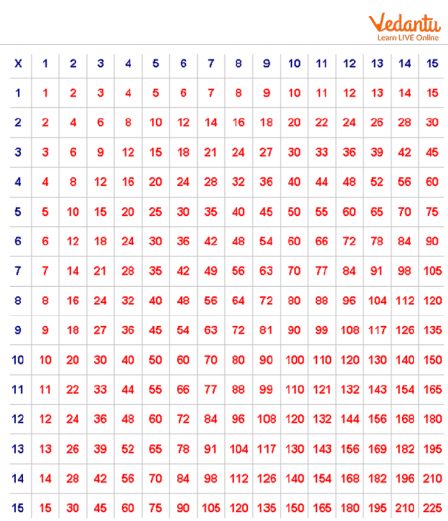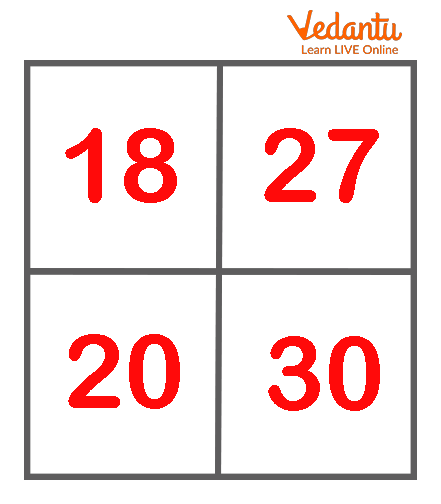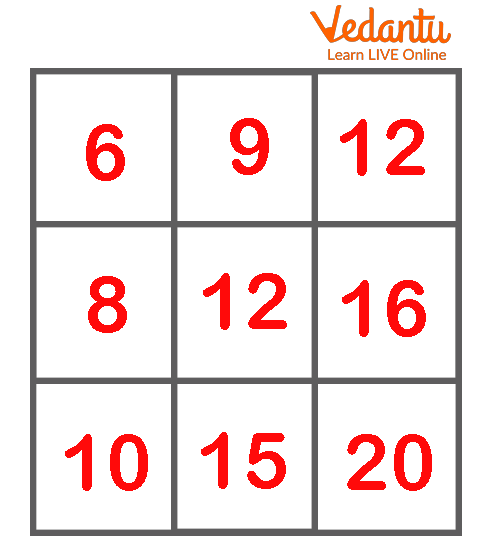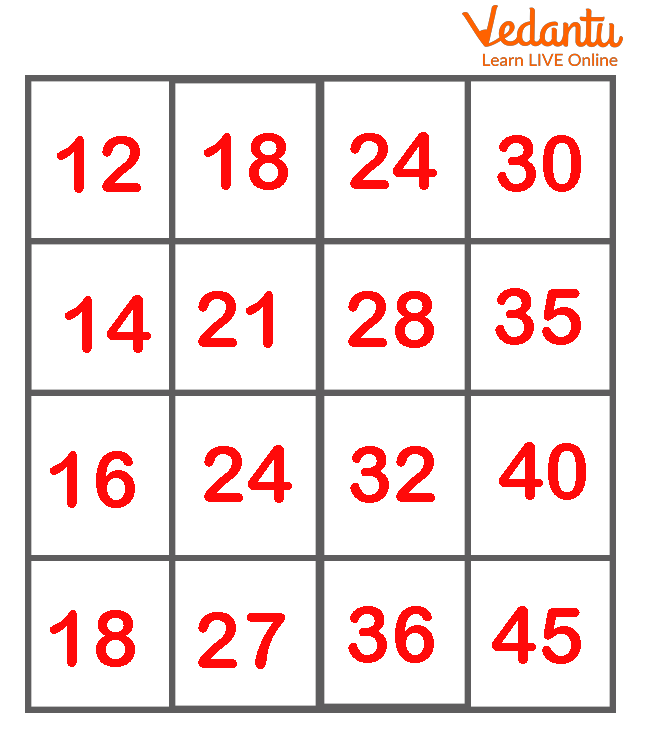Courses
Courses for Kids
Free study material
Free LIVE classes
More

# Multiplication SquaresLIVE
Join Vedantu’s FREE Mastercalss

## Understanding Multiplication with a Table

The multiplication square makes understanding multiplication tables easy. Here, we will find the answer to any product up to 12 x 12 using the grid. From the left-hand column, select the number to be multiplied, then match it with the number from the top row that you wish to multiply it by. The small square where the two numbers meet will be the product.

## Multiplication Square Grid for Kids

A number sequence grid is a grid that is filled with numbers that can help students solve a variety of mathematical sums based on multiplication or times tables. Here, we will learn about a multiplication square table which is given below.Multiplication Square Grid

For example, here if you want to find 10 x 5, you have to choose 10 from the 1st column and match it with the grid in the 1st row where we have 5. The intersection of these two is grid 50. So, the answer is 50.

## An Activity to Understand the Pattern from the Above Table

1. Pick any 2 x 2 square from the grid above and add the numbers on the diagonals. For this square, you’ll get a difference of 1 for the sums on each diagonal.Multiplication square grid of 2x2

• The sum of numbers along one diagonal will be 48 (18+30).

• The sum of numbers along the other diagonal will be 47 (20+27).

2. Now pick any 3 x 3 square from the grid above and add the numbers on the diagonals. For this square, you’ll get a difference of 4 for the sums on each diagonal.Multiplication square grid of 3x3

• The sum of numbers along one diagonal is 38 (6+12+20).

• The sum of numbers along the other diagonal is 34 (10+12+12).

3. Now pick any 4 x 4 square and add the numbers on each diagonal. For this square, you’ll get a difference of 10 for the sums on each diagonal.Multiplication square grid of 4x4

• The sum of numbers along one diagonal is 110 (12+21+32+45).

• The sum of numbers along the other diagonal is 100 (18+24+28+30).

## Importance of Multiplication Squares

• Multiplication tables improve a student's mathematical learning.

• It gives them a solid understanding of the facts related to multiplication.

• It makes it easier for kids to solve mathematical problems.

• Students who know multiplication tables fluently are more self-assured while studying new maths concepts.

## Conclusion

A number square can be used to help kids in identifying and working with number patterns. A multiplication number square is a visual aid that helps kids identify the pattern in which each set of multiples occurs. Thus the above information can be highly beneficial for students.

Last updated date: 20th Sep 2023
Total views: 132.9k
Views today: 4.32k

## FAQs on Multiplication Squares

1. What is a number square?

It's basically a square with numbers on it. Usually, the numbers are arranged sequentially. Young kids can frequently utilise a number square with numbers ranging from 1 to 20. Number squares ranging from 1 to 100 are also common. Number squares may also be referred to as a '100 grid' or a 'multiplication grid,' with the latter specifically helping students with their multiplication or times tables.

2. How does a number square help learning?

It's a simple math tool that assists children with addition, multiplication, and subtraction. The grid can be used by children to count up or down a set a couple of times, as well as to count forward to find the next multiplication or sequence of numbers and to find prime numbers. An activity can also be conducted where the squares can be shaded or coloured, making it more enjoyable for children. Number squares can also be used to help kids in pattern recognition such as sequencing.

3. Is it important to memorise multiplication tables?

Yes, knowing multiplication tables is important. We can't always use repeated addition to multiply numbers. There must be a way to find the product with less time and effort. Multiplication helps in the solution of problems involving both fractional and integer operations. Multiplication tables are effective tools for dealing with a variety of arithmetic problems.